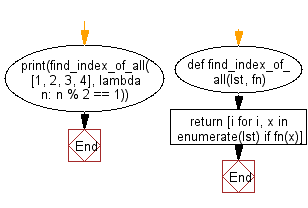﻿ Python: Find the indexes of all elements in the given list that satisfy the provided testing function - w3resource# Python: Find the indexes of all elements in the given list that satisfy the provided testing function

## Python List: Exercise - 230 with Solution

Write a Python program to find the indexes of all elements in the given list that satisfy the provided testing function.

Use enumerate() and a list comprehension to return the indexes of the all element in lst for which fn returns True.

Sample Solution:

Python Code:

``````def find_index_of_all(lst, fn):
return [i for i, x in enumerate(lst) if fn(x)]
print(find_index_of_all([1, 2, 3, 4], lambda n: n % 2 == 1))
```
```

Sample Output:

```[0, 2]
```

Flowchart:## Visualize Python code execution:

The following tool visualize what the computer is doing step-by-step as it executes the said program:

Python Code Editor:

Have another way to solve this solution? Contribute your code (and comments) through Disqus.

What is the difficulty level of this exercise?

Test your Python skills with w3resource's quiz

﻿

## Python: Tips of the Day

```print(2_000_000)
```2000000